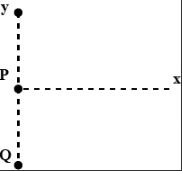Courses

# Waves MSQ

## 10 Questions MCQ Test Topic wise Tests for IIT JAM Physics | Waves MSQ

Description
This mock test of Waves MSQ for IIT JAM helps you for every IIT JAM entrance exam. This contains 10 Multiple Choice Questions for IIT JAM Waves MSQ (mcq) to study with solutions a complete question bank. The solved questions answers in this Waves MSQ quiz give you a good mix of easy questions and tough questions. IIT JAM students definitely take this Waves MSQ exercise for a better result in the exam. You can find other Waves MSQ extra questions, long questions & short questions for IIT JAM on EduRev as well by searching above.
QUESTION: 1

### Which of the below mentioned condition are necessary for beats?

Solution:

Explanation : Beats occur whenever two sound sources emit sounds of slightly different frequencies. Perhaps you recall the demonstration in class with the two tuning forks of slightly different frequencies.

*Multiple options can be correct
QUESTION: 2

### Choose the correct statements for standing waves.

Solution:

The correct answers are: There is no transport of energy along a standing wave, Each particle on a string (on which a standing wave is travelling) is oscillating with frequency common to the two superposing waves., The amplitude of each point on such string depends on the location of the point

*Multiple options can be correct
QUESTION: 3

### Choose the correct statem ents

Solution:

The correct answers are: Phase velocity can be greater than the speed of light., Phase velocity is only a mathematical concept, Phase velocity represents the speed at which a given phase of monochromatic wave train advances.

*Multiple options can be correct
QUESTION: 4

Choose the correct statements when both the source & detector are in motion

Solution:

The correct answers are: The frequency observed can be greater than the actual frequency, The frequency observed can be less than the actual frequency

*Multiple options can be correct
QUESTION: 5

Choose the correct statements

Solution:

The correct answers are: In a non dispersive medium, group velocity is equal to phase velocity., In a dispersive medium, the two velocity are not the same

QUESTION: 6

Two identical sources P and Q emit waves in phase and of same wavelength λ. Spacing between P and Q is 3λ. The maximum distance from P along the horizontal for which minimum occurs is given bySolution:
*Multiple options can be correct
QUESTION: 7

Select the correct options

Solution:

The correct answers are: Interference is superposition of travelling waves in space., Beats is superposition of travelling waves in time.

*Multiple options can be correct
QUESTION: 8

Maximum change in the frequency is observed in which of the following conditions

Solution:

The correct answers are: Source & detector move towards each other, Source & detector move away from each other

QUESTION: 9

Choose the correct options

Solution: